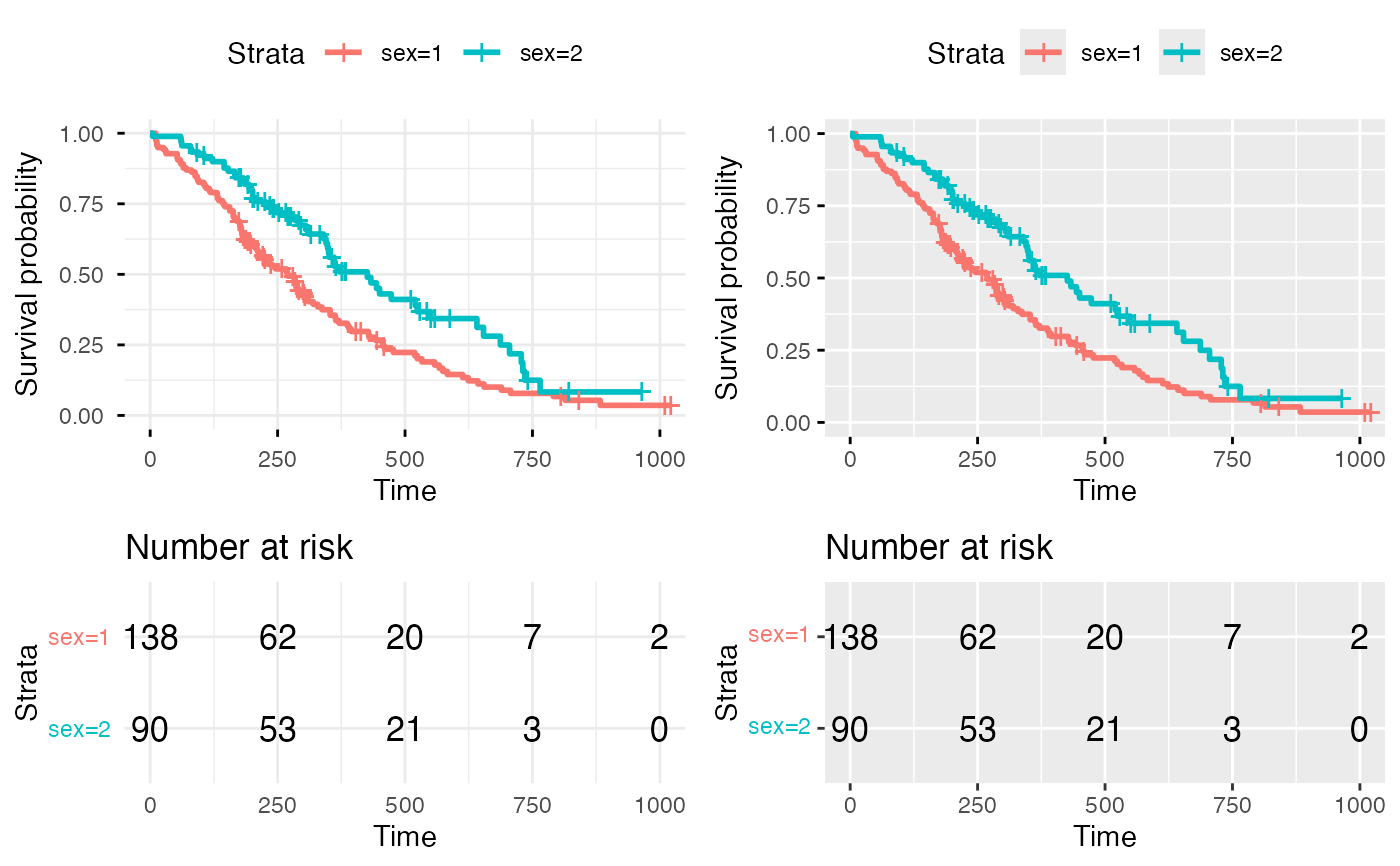Arranging multiple ggsurvplots on the same page.

arrange_ggsurvplots(
x,
print = TRUE,
title = NA,
ncol = 2,
nrow = 1,
surv.plot.height = NULL,
risk.table.height = NULL,
ncensor.plot.height = NULL,
...
)

## Arguments

x a list of ggsurvplots. logical value. If TRUE, the arranged plots are displayed. character vector specifying page title. Default is NA. the number of columns and rows, respectively. the height of the survival plot on the grid. Default is 0.75. Ignored when risk.table = FALSE. 1-risk.table.height - ncensor.plot.height when risk.table = TRUE and ncensor.plot = TRUE the height of the risk table on the grid. Increase the value when you have many strata. Default is 0.25. Ignored when risk.table = FALSE. The height of the censor plot. Used when ncensor.plot = TRUE. not used

## Value

returns an invisible object of class arrangelist (see marrangeGrob), which can be saved into a pdf file using the function ggsave.

## Examples


# Fit survival curves
require("survival")
fit<- survfit(Surv(time, status) ~ sex, data = lung)

# List of ggsurvplots
require("survminer")
splots <- list()
splots[] <- ggsurvplot(fit, data = lung, risk.table = TRUE, ggtheme = theme_minimal())#> Warning: Vectorized input to element_text() is not officially supported.
#> Results may be unexpected or may change in future versions of ggplot2.splots[] <- ggsurvplot(fit, data = lung, risk.table = TRUE, ggtheme = theme_grey())#> Warning: Vectorized input to element_text() is not officially supported.
#> Results may be unexpected or may change in future versions of ggplot2.
# Arrange multiple ggsurvplots and print the output
arrange_ggsurvplots(splots, print = TRUE,
ncol = 2, nrow = 1, risk.table.height = 0.4)if (FALSE) {
# Arrange and save into pdf file
res <- arrange_ggsurvplots(splots, print = FALSE)
ggsave("myfile.pdf", res)
}